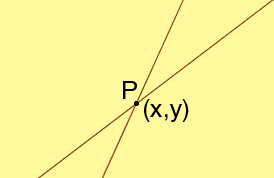y = 3x + 2 y = 4x - 5 solve for x Courtney Hi Courtney, Each of the two equations, y = 3x + 2 and y = 4x - 5 is the equation of a line in the plane. The instruction "solve for x" asks you to find the x-coordinate of the point where they intersect.If you knew the x-coordinate of this point of intersection then you could find the y-coordinate in two different ways. Since the point is on the first line the y-coordinate is given by y = 3x + 2 and since the point is on the second line the y-coordinate is given by y = 4x - 5. But this point is on both lines so the value for the y-coordinate must be the same in both cases. That is 4x - 5 = 3x + 2 Now can you solver for x? Penny Go to Math Central